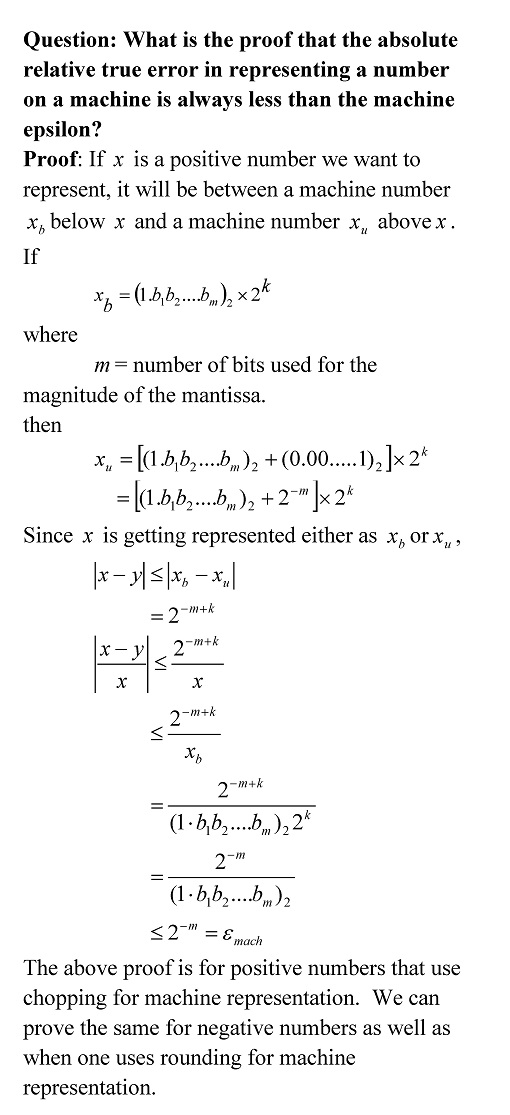## Machine epsilon – Question 5 of 5

In the previous blog posts, we answered

Here we answer the last question.• _________________

This post is brought to you by

• Holistic Numerical Methods Open Course Ware:
• the textbooks on
• the Massive Open Online Course (MOOCs) available at

## Machine epsilon – Question 3 of 5

In the previous blog posts, we answered

Here we answer the next question.Future posts will answer these questions
Question 4 of 5: What is the significance of machine epsilon for a student in an introductory course in numerical methods?

Question 5 of 5: What is the proof that the absolute relative true error in representing a number on a machine is always less than the machine epsilon?
________________________

_________________

This post is brought to you by

• Holistic Numerical Methods Open Course Ware:
• the textbooks on
• the Massive Open Online Course (MOOCs) available at

## Machine epsilon – Question 2 of 5

In the previous blog post, we answered

Here we answer the next question.Future posts will answer these questions
Question 3 of 5: How is machine epsilon related to the number of bits used to represent a floating point number?
Question 4 of 5: What is the significance of machine epsilon for a student in an introductory course in numerical methods?
Question 5 of 5: What is the proof that the absolute relative true error in representing a number on a machine is always less than the machine epsilon?

_________________

This post is brought to you by

• Holistic Numerical Methods Open Course Ware:
• the textbooks on
• the Massive Open Online Course (MOOCs) available at

## Machine epsilon – Question 1 of 5Future posts will answer these questions

Question  2 of 5: How do I find the machine epsilon using a MATLAB code?

Question 3 of 5: How is machine epsilon related to the number of bits used to represent a floating-point number?

Question 4 of 5: What is the significance of machine epsilon for a student in an introductory course in numerical methods?

Question 5 of 5: What is the proof that the absolute relative true error in representing a number on a machine is always less than the machine epsilon?

_________________

This post is brought to you by

• Holistic Numerical Methods Open Course Ware:
• the textbooks on
• the Massive Open Online Course (MOOCs) available at